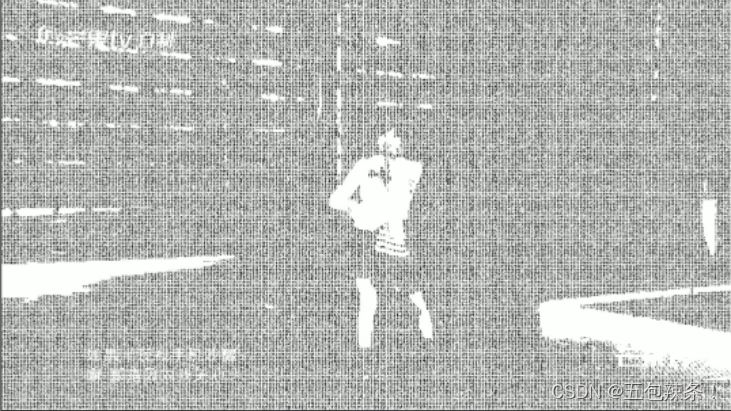# 利用Python字符畫生成甜心教主

## 項目效果展示## 項目思路解析### 1.視頻拆分成視頻

```# 將視頻轉換為圖片 並進行計數，返回總共生成瞭多少張圖片！
def video_to_pic(vp):
# vp = cv2.VideoCapture(video_path)
number = 0
if vp.isOpened():
if not os.path.exists('cache_pic'):
os.mkdir('cache_pic')
os.chdir('cache_pic')
else:
r = False
while r:
number += 1
cv2.imwrite(str(number) + '.jpg', frame)
print('\n由視頻一共生成瞭{}張圖片！'.format(number))
os.chdir("..")
return number
```

### 2.將圖片轉換字符畫

```def img2strimg(frame, K=3):
# 讀取矩陣的長度 有時返回兩個值，有時三個值
height, width, *_ = frame.shape
# print(frame.shape)
# 顏色空間轉化 圖片對象， 灰度處理
frame_gray = cv2.cvtColor(frame, cv2.COLOR_BGR2GRAY)
# print(frame_gray)
# 轉換數據類型，將數據降維
frame_array = np.float32(frame_gray.reshape(-1))
# print(frame_array)
# 得到labels(類別)、centroids(矩心) compactness(密度值)。
# 如第一行6個像素labels=[0,2,2,1,2,0],則意味著6個像素分別對應著 第1個矩心、第3個矩心、第3、2、3、1個矩心。
compactness, labels, centroids = cv2.kmeans(frame_array, K, None, (cv2.TERM_CRITERIA_EPS + cv2.TERM_CRITERIA_MAX_ITER, 10, 1.0), 10, cv2.KMEANS_RANDOM_CENTERS)
print(labels)
centroids = np.uint8(centroids)  # 轉換成整形
# labels的數個矩心以隨機順序排列，所以需要簡單處理矩心.
# 返回一個折疊成一維的數組
centroids = centroids.flatten()
# 排序
centroids_sorted = sorted(centroids)
# 獲得不同centroids的明暗程度，0最暗
centroids_index = np.array([centroids_sorted.index(value) for value in centroids])
# 亮度設置
bright = [abs((3 * i - 2 * K) / (3 * K)) for i in range(1, 1 + K)]
bright_bound = bright.index(np.min(bright))
# 背景陰影設置
shadow = [abs((3 * i - K) / (3 * K)) for i in range(1, 1 + K)]
# 返回一個折疊成一維的數組
labels = labels.flatten()
print(labels)
# 將labels轉變為實際的明暗程度列表，0最暗。
labels = centroids_index[labels]
print(labels)
# 列表解析，每2*2個像素挑選出一個，組成（height*width*灰）數組。
labels_picked = [labels[rows * width:(rows + 1) * width:2] for rows in range(0, height, 2)]
canvas = np.zeros((3 * height, 3 * width, 3), np.uint8)
canvas.fill(255)  # 創建長寬為原圖三倍的白色畫佈。

# 因為 字體大小為0.45時，每個數字占6*6個像素，而白底畫佈為原圖三倍
# 所以 需要原圖中每2*2個像素中挑取一個，在白底畫佈中由6*6像素大小的數字表示這個像素信息。
y = 0
for rows in labels_picked:
x = 0
for cols in rows:
# 添加文字  圖片，添加的文字，左上角坐標，字體，字體大小，顏色，字體粗細
cv2.putText(canvas, str(random.randint(2, 9)), (x, y), cv2.FONT_HERSHEY_PLAIN, 0.45, 0.1)
elif cols <= bright_bound:
cv2.putText(canvas, "-", (x, y),cv2.FONT_HERSHEY_PLAIN, 0.4, 0, 1)
x += 6
y += 6

return canvas
```

### 3.合成視頻

```def jpg_to_video(char_image_path, FPS):
video_fourcc = cv2.VideoWriter_fourcc(*"MP42")  # 設置視頻編碼器,這裡使用使用MP42編碼器,可以生成更小的視頻文件
char_img_path_list = [char_image_path + r'/{}.jpg'.format(i) for i in range(1, number + 1)]  # 生成目標字符圖片文件的路徑列表
char_img_test = Image.open(char_img_path_list).size  # 獲取圖片的分辨率
if not os.path.exists('video'):
os.mkdir('video')
video_writter = cv2.VideoWriter('video/new_char_video.avi', video_fourcc, FPS, char_img_test)
sum = len(char_img_path_list)
count = 0
for image_path in char_img_path_list:
video_writter.write(img)
end_str = '100%'
count = count + 1
process_bar(count / sum, start_str='', end_str=end_str, total_length=15)

video_writter.release()
print('\n')
print('=======================')
print('The video is finished!')
print('=======================')

```

## 簡易源碼分享

```# from platypus import
import os
from PIL import Image, ImageFont, ImageDraw
import cv2
import random
import numpy as np

# 將視頻轉換為圖片 並進行計數，返回總共生成瞭多少張圖片！
def video_to_pic(vp):
# vp = cv2.VideoCapture(video_path)
number = 0
if vp.isOpened():
if not os.path.exists('cache_pic'):
os.mkdir('cache_pic')
os.chdir('cache_pic')
else:
r = False
while r:
number += 1
cv2.imwrite(str(number) + '.jpg', frame)
print('\n由視頻一共生成瞭{}張圖片！'.format(number))
os.chdir("..")
return number

def star_to_char(number, save_pic_path):
if not os.path.exists('cache_char'):
os.mkdir('cache_char')
img_path_list = [save_pic_path + r'/{}.jpg'.format(i) for i in range(1, number + 1)]  # 生成目標圖片文件的路徑列表
for image_path in img_path_list:
img_width, img_height = Image.open(image_path).size  # 獲取圖片的分辨率
print('=======================')
print('All image was finished!')
print('=======================')
return 0

def img2strimg(frame, K=3):
# 讀取矩陣的長度 有時返回兩個值，有時三個值
height, width, *_ = frame.shape
# print(frame.shape)
# 顏色空間轉化 圖片對象， 灰度處理
frame_gray = cv2.cvtColor(frame, cv2.COLOR_BGR2GRAY)
# print(frame_gray)
# 轉換數據類型，將數據降維
frame_array = np.float32(frame_gray.reshape(-1))
# print(frame_array)
# 得到labels(類別)、centroids(矩心) compactness(密度值)。
# 如第一行6個像素labels=[0,2,2,1,2,0],則意味著6個像素分別對應著 第1個矩心、第3個矩心、第3、2、3、1個矩心。
compactness, labels, centroids = cv2.kmeans(frame_array, K, None, (cv2.TERM_CRITERIA_EPS + cv2.TERM_CRITERIA_MAX_ITER, 10, 1.0), 10, cv2.KMEANS_RANDOM_CENTERS)
print(labels)
centroids = np.uint8(centroids)  # 轉換成整形
# labels的數個矩心以隨機順序排列，所以需要簡單處理矩心.
# 返回一個折疊成一維的數組
centroids = centroids.flatten()
# 排序
centroids_sorted = sorted(centroids)
# 獲得不同centroids的明暗程度，0最暗
centroids_index = np.array([centroids_sorted.index(value) for value in centroids])
# 亮度設置
bright = [abs((3 * i - 2 * K) / (3 * K)) for i in range(1, 1 + K)]
bright_bound = bright.index(np.min(bright))
# 背景陰影設置
shadow = [abs((3 * i - K) / (3 * K)) for i in range(1, 1 + K)]
# 返回一個折疊成一維的數組
labels = labels.flatten()
print(labels)
# 將labels轉變為實際的明暗程度列表，0最暗。
labels = centroids_index[labels]
print(labels)
# 列表解析，每2*2個像素挑選出一個，組成（height*width*灰）數組。
labels_picked = [labels[rows * width:(rows + 1) * width:2] for rows in range(0, height, 2)]
canvas = np.zeros((3 * height, 3 * width, 3), np.uint8)
canvas.fill(255)  # 創建長寬為原圖三倍的白色畫佈。

# 因為 字體大小為0.45時，每個數字占6*6個像素，而白底畫佈為原圖三倍
# 所以 需要原圖中每2*2個像素中挑取一個，在白底畫佈中由6*6像素大小的數字表示這個像素信息。
y = 0
for rows in labels_picked:
x = 0
for cols in rows:
# 添加文字  圖片，添加的文字，左上角坐標，字體，字體大小，顏色，字體粗細
cv2.putText(canvas, str(random.randint(2, 9)), (x, y), cv2.FONT_HERSHEY_PLAIN, 0.45, 0.1)
elif cols <= bright_bound:
cv2.putText(canvas, "-", (x, y),cv2.FONT_HERSHEY_PLAIN, 0.4, 0, 1)
x += 6
y += 6

return canvas

def jpg_to_video(char_image_path, FPS):
video_fourcc = cv2.VideoWriter_fourcc(*"MP42")  # 設置視頻編碼器,這裡使用使用MP42編碼器,可以生成更小的視頻文件
char_img_path_list = [char_image_path + r'/{}.jpg'.format(i) for i in range(1, number + 1)]  # 生成目標字符圖片文件的路徑列表
char_img_test = Image.open(char_img_path_list).size  # 獲取圖片的分辨率
if not os.path.exists('video'):
os.mkdir('video')
video_writter = cv2.VideoWriter('video/new_char_video.avi', video_fourcc, FPS, char_img_test)
sum = len(char_img_path_list)
count = 0

if __name__ == '__main__':
video_path = '王心凌.mp4'
save_pic_path = 'cache_pic'
save_charpic_path = 'cache_char'
vp = cv2.VideoCapture(video_path)
number = video_to_pic(vp)
for i in range(1, number):
fp = r"cache_pic/{}.jpg".format(i)
img = cv2.imread(fp)  # 返回圖片數據 （高度， 寬度，通道數）
print(img)
# 若字符畫結果不好，可以嘗試更改K為3。若依然無法很好地表現原圖，請換圖嘗試。 -_-||
str_img = img2strimg(img)
cv2.imwrite("cache_char/{}.jpg".format(i), str_img)
# number = 1692
# print(number)
FPS = vp.get(cv2.CAP_PROP_FPS)
star_to_char(number, save_pic_path)
jpg_to_video(save_charpic_path, FPS)
```